19.1 numbers- introduction

We have three types of numbers, cardinal numbers, ordinal numbers and fraction numbers.

Cardinal numbers are simple from a linguistic point of view. They just describe the amount of something. Four apples is more than three apples, that's very obvious.

Much more complicated from a linguistic point of view are ordinal numbers. Ordinal numbers don't describe the amount of something but the position of an element of a group in relation to another element of a group. If someone tells us that the third apple from the left is red we know which apple is red but we don' t know how many apple there are. From a linguistic point of view ordinal numbers are complicated because they are nouns, in other words they have feminine / masculine, a singular / plural form (the first / the firsts) and they can be the subject of a sentence and a indirect / direct object.

Fraction numbers describe the amount of fractions of an entity. Three parts of a fifth means 3/5 * 100 = 60 per cent. Fifth is the entire unity and we have three times one part of this entity.

 contact privacy statement imprint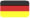GERMAN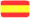SPANISH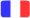FRENCH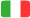ITALIAN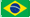PORTUGUESE